## Introduction to Line Integrals

Consider the following problem: a piece of string, corresponding to a curve C, lies in the xy-plane. The mass per unit length of the string is f(x,y). What is the total mass of the string?

The formula for the mass is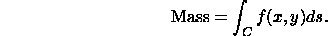The integral above is called a line integral of f(x,y) along C. It is also called a line integral with respect to arc length.

Question: how do we actually evaluate the above integral? The strategy is: (0) parameterize the curve C, (1) cut up the curve C into infinitesimal pieces, (2) determine the mass of each infinitesimal piece, (3) integrate to determine the total mass. It is assumed that C is piecewise smooth. That is, it is a union of finite number of smooth curves.

Suppose that we can describe the curve by the vector function r(t)=<x(t),y(t)> where a<=t<=b. Consider a portion of the curve corresponding to the infinitesimal interval t_0<=t<=t_0+dt.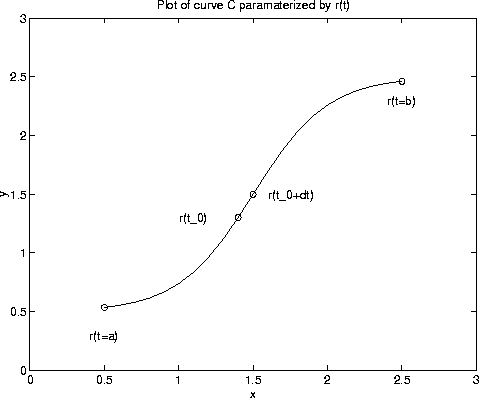The arc length of the curve, ds, on this interval is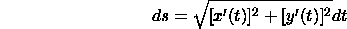Hence, the mass of the piece (density times length) isThe total mass of the string is the sum of all the masses of all infinitesimal piecesThe integral on the right is an integral of one variable.

Line integrals are not restricted to curves in the xy plane. If C is a curve in three dimensions parameterized by r(t)=<x(t),y(t),z(t)> with a<=t<=b, thenFind the mass of the piece of wire described by the curve x^2+y^2=1 with density function f(x,y)=3+x+y.

The circle of radius 1 can be parameterized by the vector function r(t)=<cos(t),sin(t)> with 0<=t<=2*pi. We have x(t)=cos(t) and y(t)=sin(t), so x'(t)=-sin(t) and y'(t)=cos(t). The mass is given by the formula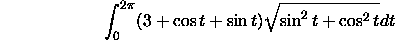The term in the square root is 1, hence we have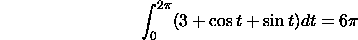In some applications, such as line integrals of vector fields, the following line integral with respect to x arises:This is an integral over some curve C in xyz space. It can be converted to integral in one variable. Suppose that C can be parameterized by r(t)=<x(t),y(t),z(t)> with a<=t<=b. Then,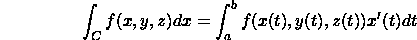There are analogous formulas for integrals with respect to y and z.

In some applications, integrals with respect to x, y, and z occur in a sum:If C is a curve in the xy plane and R=0,  it might be possible to evaluate the line integral using Green's theorem.

Using the standard parameterization for C, this last integral becomes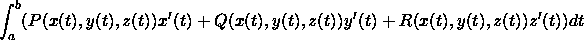Evaluate the line integralwhere C is the circle in the figure above.

Recall that the circle is parameterized by r(t)=<cos(t),sin(t)> with 0<=t<=2*pi. Hence, x(t)=cos(t), y(t)=sin(t), and y'(t)=cos(t). According to the discussion above,[Vector Calculus Home] [Math 254 Home] [Math 255 Home] [Notation] [References]